# YruRU Method##### (U) R U2 R' U' R U' R' U2 R' U2 R U R' U R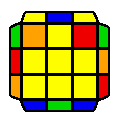##### R2 U R2 U2 R' U' R U' R U' R U' R2 U2 R'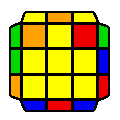##### R' U' R U' R' U2 R2 U R' U R U2 R'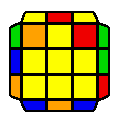##### (U2) R U R' U R U2 R2 U' R U' R' U2 R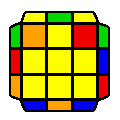##### (U2) R U R' U R' U2 R2 U R2 U R2 U' R'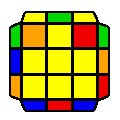##### R' U' R U' R U2 R2 U' R2 U' R2 U R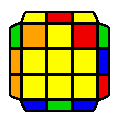##### (U) R U' R U R U' R' U' R U2 R' U2 R' U R'##### (U) R U2 R' U' R U' R' U' R U R' U R U2 R'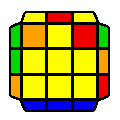##### (U') R' U' R U R' U R U2 R' U R U2 R' U' R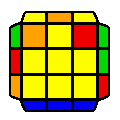##### R' U' R U' R' U2 R U' R' U2 R U R' U R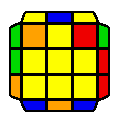##### (U) R' U2 R U R' U R U R' U' R U' R' U2 R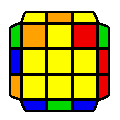##### (U) R U2 R2 U' R2 U' R' U R' U' R U R' U R

Average movecount: 14.3

Average double turns: 2.8

Algorithms: 12

If there is a 2x3 block, then it is a 2-twist case, which is simply 2 sune algorithms connected with a U move. If there is a 1x3 block (2 cases), then the case can be identified depending on the position of the block on the side.

If there are no blocks (3 cases), then the 2x3 area may look like an H perm, or there may be a checker-board pattern extending to either right, or left.

If there are two blocks (2 cases) they will be parallel, and their algorithms are simply 2 sune algorithms connected with a U move.

If there is a single block (4 cases), then the case can be decided based on the position of the block. If the block is in the back half of side, then the algorithm is simply 2 sune algorithms with two R moves fused as an R2.

Note, these rules are only to make learning of these cases simpler. For speed, a better recognition system is to be adopted.